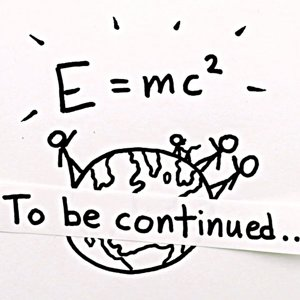# E=mc2 Definition and 10 Discussions

In physics, mass–energy equivalence is the relationship between mass and energy in a system's rest frame, where the two values differ only by a constant and the units of measurement. The principle is described by the physicist Albert Einstein's famous formula:

The formula defines the energy E of a particle in its rest frame as the product of mass (m) with the speed of light squared (c2). Because the speed of light is a large number in everyday units (approximately 3×108 meters per second), the formula implies that a small amount of rest mass corresponds to an enormous amount of energy, which is independent of the composition of the matter. Rest mass, also called invariant mass, is the mass that is measured when the system is at rest. It is a fundamental physical property that is independent of momentum, even at extreme speeds approaching the speed of light (i.e., its value is the same in all inertial frames of reference). Massless particles such as photons have zero invariant mass, but massless free particles have both momentum and energy. The equivalence principle implies that when energy is lost in chemical reactions, nuclear reactions, and other energy transformations, the system will also lose a corresponding amount of mass. The energy, and mass, can be released to the environment as radiant energy, such as light, or as thermal energy. The principle is fundamental to many fields of physics, including nuclear and particle physics.
Mass–energy equivalence arose from special relativity as a paradox described by the French polymath Henri Poincaré. Einstein was the first to propose the equivalence of mass and energy as a general principle and a consequence of the symmetries of space and time. The principle first appeared in "Does the inertia of a body depend upon its energy-content?", one of his Annus Mirabilis (Miraculous Year) papers, published on 21 November 1905. The formula and its relationship to momentum, as described by the energy–momentum relation, were later developed by other physicists.

View More On Wikipedia.org
1. ### I Why does E=mc²?

Sean Carroll says that in SR the time component of the 4-momentum of a particle is its energy. It is of course also ##mc^2dt/d\tau##. He uses that to prove that ##E=mc^2##. Which begs the question why does ##E=p^0##? Misner, Thorne, Wheeler do roughly the same thing. I find these 'proofs'...
2. ### B Where does the increase in mass come from in fission?

If energy is released during nuclear fission, why is the mass of the products (the two new nuclei and fission neutrons) greater than the mass of the original nuclei? In accordance to E=mc^2, shouldn't the release of energy result in the products having a lesser mass than the original nuclei?
3. ### I Subbing Planck length into length contraction equation?

I was wondering if it is possible to work out the maximum amount of energy an object with mass can have using the length contraction equation (i.e. "actual" length divided by Lorentz factor). The way I thought of doing this was by rearranging e = mc^2 to get c^2 = e/m. Then, substitute e/m into...
4.### E=mc² is Incomplete

This is a video of one of our most frequently-asked question, and frequently misunderstood concept.
5. ### Bohr model and relativistic electron mass

From the semi-classical Bohr model of the hydrogen atom the velocity of the electron in a certain orbit can be determined. With these velocities the electron's relativistic masses can be determined. With E=mc2 the energy levels are in agreement with those from the Bohr model. I know Bohr's model...
6. ### Matter conversion into power

Homework Statement What mass of mater would be converted to energy to provide 3200W of Power for 3 days? Homework Equations P=E/t E=mc2 The Attempt at a Solution [/B] E=P*t Subbing mc2 for energy I get mc2=P*t m=P*t/c2 m=(3200W*3Days)/(9X1016m2/s2) m= 1.066X10-13kg This answer is...
7. ### E=mc^2 vs energy in strong interaction

Hi everyone, I have a simple and foolish question. I want to compare the energy of a given mass (obviously e=mc2); let's say the energy of a hydrogen atom, with the energy that binds together the fundamental particles of that atom (strong interaction). I know that e=mc2 holds always true, and...
8. ### Photon Mass in E=MC^2

A photon has “zero” rest mass, but it does have Energy, But with zero Mass , E=MC^2 yields E=0 I am confused !